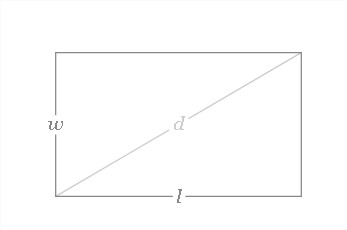# C Program To Calculate Perimeter Of Rectangle | C Programs

C Program to Calculate the perimeter of a rectangle – In this distinct article, we will brief in on all the ways you can calculate the perimeter of a rectangle in C Programming.

The ways that are used to do so in this blog are as follows:

• Using Standard Method
• Using Functions
• Using Pointers
• Using Macros

In this piece, we have added sample programs and suitable examples for your easy understanding. The compiler has been added as well for a better understanding.

As we all know, a rectangle is a 2-dimensional quadrilateral figure whose opposite sides are equal and parallel. All the angles of a rectangle are also equal to 90 degrees.

A rectangle looks like this:As you can see, since the opposite sides of a rectangle are equal, the perimeter of a rectangle can be calculated with this formula:

Perimeter = l + b + l + b = 2 (l + b)

The multiple methods mentioned are as follows:

## Using Standard Method

1)The formula for the perimeter of a rectangle is perimeter=2*(length+breadth)

2)We are calculating the perimeter by substituting the length and breadth values into related variables “length”, “breadth”, the calculated value will store into “perimeter” variable.

output:

### Using Functions

1)Here we are using the function float perimeter(float l,float b) to calculate perimeter,which returns the float value.

2)We are calling the function using perimeter(l,b)where l,b are float type arguments

3)float perimeter(float l, float b) calculate the perimeter and return the value, that value will store into variable “p”

output:

#### Using Pointers

1)Here  *p,*l,*b are pointer variables

2)we are calling the function using perimeter(&l,&b,&p), here we are passing addresses

3)void perimeter(float *l,float *b,float *p) function calculate the perimeter ,the value will store into pointer variable *p

output:
##### Using Macros

1)The formula for the perimeter of rectangle 2*(l+b) was defined with the name perimeter(l,b).

2)perimeter(l,b)replaced with that expression given at #define.

output:
x

## C Program Inverted Pyramid Star Pattern | 4 Ways – C Programs

C Program to print an inverted pyramid star pattern – In this article, we will ...